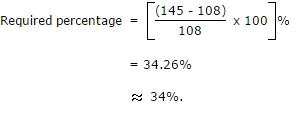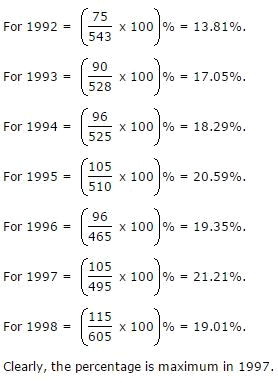# Important DI – Table  for SBI PO Mains – 2017

Directions (1- 5): Study the given chart carefully and answer the following questions.

Train A:

 Station Arrival Time Departure Time Distance From orign in Kms No. of passenger boarding at each station Fare in Rs Ahmedabad Starting 5.00 pm — 400 — Vadodara 6.30 pm 6.35 pm 100 100 50 Bharuch 8.50 pm 9.00 pm 250 90 120 Mumbai 4.00 am 4.10 am 800 300 400 Pune 7.30 am 7.45 am 1050 150 500 Solapur 10.20 am Terminates 1280 — 620

Train B:

 Station Arrival Time Departure Time Distance From orign in Kms No. of passenger boarding at each station Fare in Rs Solapur Starting 6.00 pm — 300 — Pune 7.40 pm 7.45 pm 230 150 120 MUmbai 9.30 pm 9.35 pm 480 270 220 Bharuch 5.40 am 5.55 am 1030 50 500 Vadodara 9.00 am 9.10 am 1180 100 570 Ahmedbad 12.00 noon Terminates 1280 — 620

1. The number of passengers boarding Train A at Vadodara is what percent of the number of passengers boarding Train B at Mumbai?

a) 37.03%

b) 47.03%

c) 27.03%

d) 47.30%

e) None of these

a) 37.03%

Required percentage = 100/270 × 100 = 37.03%

2. What is the difference between the speed of Train A and that of Train B?

(a) 2.73 kmph

(b) 1.97 kmph

(c) 3.6 kmph

(d) 2.62 kmph

(e) 3.9 kmph

(a) 2.73 kmph

Speed of Train A = 1280 / 10:20 am – 5:00 pm

= 1280 / 17 hours 20 minutes
= 1280 x 3 / 52 = 73.84 kmph
Speed of train B = 1280 / 12:00 noon ⎯ 6:00 pm
= 1280/18 hours = 71.11 kmph
So, difference between the speed of train A and train B = 73.84 ⎯ 71.11 = 2.73 kmph

3. What is the ratio of the total passengers of Train A to that of Train B?

(a) 102 : 79

(b) 104 : 87

(c) 103 : 87

(d) 110 : 79

(e) 113 : 87

(c) 103 : 87

Total passengers in train A = 400 + 100 + 90 + 300 + 150 = 1040
Total passengers in train B = 300 + 150 + 270 + 50 + 100 = 870
So, required ratio = 1040 : 870 = 104 : 87

4. The total income of Train A is what percent of the total income of Train B?

(a) 180%

(b) 159.51%

(c) 123.29%

(d) 125%

(e) 127.64%

(e) 127.64%

Total income of train A = (400 × 50) + (500 × 70) + (590 × 280) + (890 × 100) + (1040 × 120) = Rs.434000
Total income of train B = (300 × 120) + (450 × 100) + (620 × 280) + (670 × 70) + (770 × 50) = Rs.340000
So, required % = 434000 x 100 / 340000
= 127.64% of the total income of train B.

5. If the average speed of Train A increases by 10% then when will it reach to its destination?

(a) 7:45 am

(b) 9:45 am

(c) 8:45 am

(d) 10:45 am

(e) 11:45 am

(c) 8:45 am

If the average speed of train A increases by 10%
then its new speed = 73.84 × 110/100
= 81.22 kmph
Time taken by train A during the journey = 1280/81.22 = 15.75 hours = 15 hours 45 minutes
The time when the train will reach its destination = 5 pm + 15 hours 45 minutes = 8:45 am

Directions (6 – 10): Number of Different Types of Batteries Sold by a Company over the Years (Numbers in Thousands)

 Year Types of Batteries 4AH 7AH 32AH 35AH 55AH Total 1992 75 144 114 102 108 543 1993 90 126 102 84 126 528 1994 96 114 75 105 135 525 1995 105 90 150 90 75 510 1996 90 75 135 75 90 465 1997 105 60 165 45 120 495 1998 115 85 160 100 145 605

6. What was the approximate percentage increase in the sales of 55AH batteries in 1998 compared to that in 1992?

(a) 28%

(b) 31%

(c) 33%

(d)34%

(e)None of these

(d)34%7. The total sales of all the seven years is the maximum for which battery?

(a) 4AH

(b) 7AH

(c) 32AH

(d) 35AH

(e) None of these

(c) 32AH

The total sales (in thousands) of all the seven years for various batteries are:

For 4AH = 75 + 90 + 96 + 105 + 90 + 105 + 115 = 676

For 7AH = 144 + 126 + 114 + 90 + 75 + 60 + 85 = 694

For 32AH = 114 + 102 + 75 + 150 + 135 + 165 + 160 = 901

For 35AH = 102 + 84 + 105 + 90 + 75 + 45 + 100 = 601

For 55AH = 108 + 126 + 135 + 75 + 90 + 120 + 145 = 799.

Clearly, sales are maximum in case of 32AH batteries.

8. What is the difference in the number of 35AH batteries sold in 1993 and 1997?

(a) 24000

(b) 28000

(c) 35000

(d) 39000

(e) None of these

(d) 39000

Required difference = [(84 – 45) x 1000] = 39000.

9. The percentage of 4AH batteries sold to the total number of batteries sold was maximum in the year?

(a) 1994

(b) 1995

(c) 1996

(d) 1997
(e) None of these

(d) 1997

The percentages of sales of 4AH batteries to the total sales in different years are:10. In case of which battery there was a continuous decrease in sales from 1992 to 1997?

(a) 4AH

(b) 7AH

(c) 32AH

(d) 35AH

(e) None of these